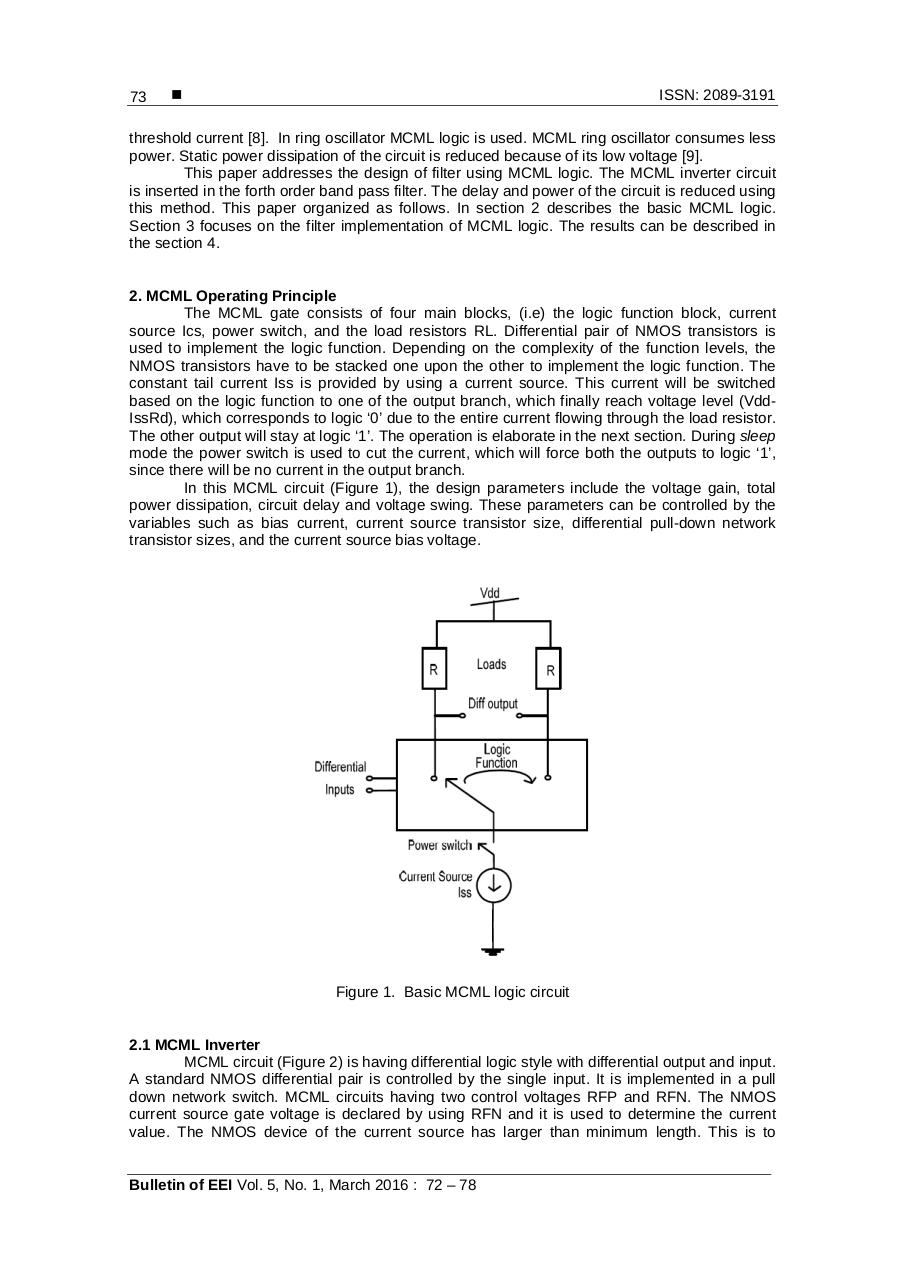# 08 458 704 1 SM.pdfPage 1 2 3 4 5 6 7

#### Text preview

73

ISSN: 2089-3191

threshold current . In ring oscillator MCML logic is used. MCML ring oscillator consumes less
power. Static power dissipation of the circuit is reduced because of its low voltage .
This paper addresses the design of filter using MCML logic. The MCML inverter circuit
is inserted in the forth order band pass filter. The delay and power of the circuit is reduced using
this method. This paper organized as follows. In section 2 describes the basic MCML logic.
Section 3 focuses on the filter implementation of MCML logic. The results can be described in
the section 4.

2. MCML Operating Principle
The MCML gate consists of four main blocks, (i.e) the logic function block, current
source Ics, power switch, and the load resistors RL. Differential pair of NMOS transistors is
used to implement the logic function. Depending on the complexity of the function levels, the
NMOS transistors have to be stacked one upon the other to implement the logic function. The
constant tail current Iss is provided by using a current source. This current will be switched
based on the logic function to one of the output branch, which finally reach voltage level (VddIssRd), which corresponds to logic ‘0’ due to the entire current flowing through the load resistor.
The other output will stay at logic ‘1’. The operation is elaborate in the next section. During sleep
mode the power switch is used to cut the current, which will force both the outputs to logic ‘1’,
since there will be no current in the output branch.
In this MCML circuit (Figure 1), the design parameters include the voltage gain, total
power dissipation, circuit delay and voltage swing. These parameters can be controlled by the
variables such as bias current, current source transistor size, differential pull-down network
transistor sizes, and the current source bias voltage.

Figure 1. Basic MCML logic circuit

2.1 MCML Inverter
MCML circuit (Figure 2) is having differential logic style with differential output and input.
A standard NMOS differential pair is controlled by the single input. It is implemented in a pull
down network switch. MCML circuits having two control voltages RFP and RFN. The NMOS
current source gate voltage is declared by using RFN and it is used to determine the current
value. The NMOS device of the current source has larger than minimum length. This is to

Bulletin of EEI Vol. 5, No. 1, March 2016 : 72 – 78